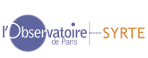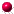BACK
EARTH ORIENTATION IN THE INTERNATIONAL REFERENCE SYSTEMS
CONSISTENCY OF IERS RESULTSREFERRING THE IERS (1976) PRECESSION-NUTATION MODEL TO THE ICRS

The IERS Conventions (2003) provide the definition of the International Celestial Reference System, the ICRS (Chapter 1), as well as procedures to be used to transform the celestial reference frame to the terrestrial one (Chapter 5). Chapter 5 also specifies a nutation model and improved numerical values for the precession rate of the equator in longitude and in obliquity.

The nutation model and the rate corrections are referred to the mean pole at J2000.0. As explained in Chapter 1, the mean pole at J2000.0 is slightly offset from the ICRS pole. In order to refer the IERS nutation model to the ICRS pole, we must therefore use additive constants in longitude and obliquity. This means that, after calculating the total displacement of the celestial pole using the corrected precession rate and the improved nutation model, a bias in both longitude and obliquity must be added in order to refer to the ICRS pole.

An estimation of the location of the mean pole at J2000.0 is presented p. 86. Based on this estimation, the constant terms to use with the IERS (1996) precession-nutation model are as follows :

In longitude: dpsi_0 = - 0.0431" In obliquity: deps_0 = - 0.0050"GLOBAL CONSISTENCY OF IERS EARTH ORIENTATION WITH THE ITRS AND THE ICRS

When IERS started operation in 1988, the EOP series were set in bias so as to be consistent with the initial realization of the International Terrestrial Reference System ITRS, the International Terrestrial Reference Frame ITRF_0, and with the ICRS. This was realized by taking advantage of the internal consistency of a selection of VLBI and SLR solutions. The uncertainty of the tie was estimated to 1-2 mas, mainly due to the poor density and geographical distribution of the colocation sites monitored by VLBI and SLR networks at this time. The time evolution of the EOP series was attached to a geological plate motion model. The uncertainty of the tie was believed to be of the order 0.1 mas/year. Therefore it could be expected that, with the increase and improvement of the data available to IERS, mas-size discrepancies would appear. These inconsistencies were monitored, with improved precision resultinmg from the increase of the overall quality of the results. In particular, the pole coordinates biases became clear when the GPS analysis centres attached their solutions to the ITRF from 1992 on. As these discrepancies were getting reasonably well understood on the one hand, and as on the other hand they had started to become misleading for high accuracy users, the Directing Board decided that the IERS EOP series would be reset in bias and rate in order to match the ITRF and ICRF. The correction was applied starting with 1 January 1997 (see IERS Gazette 8) . All past IERS series were recomputed and redistributed in the new system. The change in UT1 was complemented in order to adopt a more complete and accurate definition of GST, as recommended in the IERS Conventions (see IERS Gazette 16). The 1996 IERS Annual Report gives evaluations of the consistency of EOP with ITRS and ICRS after these changes were made. Based on these analyses, an estimation of the residual inconsistency of EOP(IERS) with the international reference systems is given in the following table.

```
CONSISTENCY OF THE IERS EOP SERIES WITH THE IERS REFERENCE FRAMES
The value to add to EOP(IERS) time series in order to make them consistent
with the ICRS and the ITRS is A+A' (t-1993.0), t in Besselian years.
-----------------------------------------------------------------------------------
Cor         x             y           UT1              dPsi         dEpsilon
0.001"        0.001"      0.0001s           0.001"         0.001"
-----------------------------------------------------------------------------------
A    +0.13 +/-0.20  -0.02 +/-0.20  -0.10 +/-0.20   +0.07 +/-0.03  -0.01 +/-0.02

A'   +0.03 +/-0.10  +0.02 +/-0.10   0.00 +/-0.10         -            -
-----------------------------------------------------------------------------------
```

###CONSISTENCY OF IERS EOP SERIES WITH ITRS AND ICRS AT A REFERENCE EPOCH

Let us consider two series of EOP (x, y, UT1, dpsi, deps and (x', y', UT1', dpsi', deps') respectively. Each of them is referred to a celestial frame defined by the adopted Radio Source Coordinates (RSC) and to a terrestrial frame defined by the adopted Set of Station Coordinates (SSC). The celestial reference frames, respectively denoted CRF and CRF' present systematic differences, which can be described by an elementary rotation matrix A. The coordinates in both system, [CRF] and [CRF'] respectively, are linked by the relation :

[CRF] = A [CRF']

In the same way the terrestrial reference frames, TRF and TRF' respectively, are linked through an elementary rotation :

[TRF] = R [TRF']

If the EOP series were consistent, then the systematic differences in the pole coordinates (Dx = x' - x, Dy = y' - y), in the universal time (DUT1 = UT1' - UT1), and in the celestial pole offsets (Dpsi = dpsi' - dpsi, Deps = deps'-deps) would be only due to the rotation angles (A1, A2, A3) between the two celestial frames and (R1, R2, R3) between the two terrestrial frames. It can be shown that these systematic differences would satisfy the following relationships with an accuracy of a few microarcseconds :

Dx = R2

Dy = R1

f. DUT1 = -R3 + A3 (1)

Dpsi = A2/sin(epsilon_0)

Deps = -A1

where f is the conversion factor from Universal Time to sidereal time, and deps is the obliquity of the ecliptic.

It is worthwhile to know to which extent the relationships (1) are satisfied. Each comparison will be made naturally with respect to the same data set (CRF, TRF, EOP), that is the ICRF, the ITRF and the corresponding EOP series of the IERS.

The closure of relationships (1) characterises the internal consistency of the set of IERS results, time series and reference frames. In this objective the quantities C(x) = Dx - R2 , C(y) = Dy - R1, C(UT1) = f.DUT1 - (-R3 + A3), C(dpsi) = Ddpsi -A2/sin(epsilon_0), C(deps) = Ddeps -(-A1) are computed.TIME EVOLUTION OF THE IERS EOP SERIES RELATIVE TO ITRF

We consider two terrestrial frames, each one having its own velocity field. The corresponding series of EOP, the relative drifts, between the series of EOP can be predicted by relationships (2), obtained as the time derivatives of the first three relationships (1):

d(Dx)/dt = d(R2)/dt ; d(Dx)/dt = d(R1)/dt ; f d(DUT1)/dt = -d(R3)/dt (2)

where d(R1)/dt, d(R2)/dt, d(R3)/dt are the rates of change of the rotation angles between the two terrestrial reference frames. These relationships are used to compare the drifts of the EOP series relative to EOP(IERS) C 01 with their predicted values based on the definition of the corresponding terrestrial velocity field.RESULTS FOR THE EOP SERIES (1999)

Since the year 1998, the angles between the individual TRF and the ITRF97 have not been computed by the terrestrial frame section of the Central Bureau (located at the Institut GŽographique National, France) for some technical reason. In turn consistency analyses of the series received during the years 1999 and 2000 cannot be made for polar motion and UT1, as the angles R1, R2, R3 are not available for computing the corresponding closure relations. However, the analysis can be restricted to the nutation offsets, as provided by VLBI techniques.

The present consistency analysis consists in two steps. The first step is the computation of the biases and slopes of the individual EOP parameters (nutation in longitude, nutation in obliquity) with respect to the combined parameters of the series EOP(IERS) C 01. The second step is the comparison, given hereafter, between these biases and slopes, and those theoritically produced by the rotations between the reference frames, as already explained.

The Table here-below gives the evaluations of the internal consistency for data sets involving celestial pole offsets series and RSC, till the date 2000.0. The celestial frames angles can be found in this Annual Report.

CONSISTENCY OF VLBI EOP SERIES WITH CELESTIAL REFERENCE FRAMES, BASED ON THE ROTATION ANGLES RELATIVE TO ICRF (Table VI-3,Annual report 1999 p. 89) at the epoch 1997 listed for dpsi, deps. The data span is given. Unit are 0.001 ''.

`---------------------------------------------------------		  1 A2/sin(epsilon_0) -A1SERIES	          2	 Ddpsi          Ddeps			  3	 C(dpsi) 	C(deps)---------------------------------------------------------		  1  .606 ± .274 -4.273 ± .143`
`EOP(GAOUA) 0 R 01 2 -.107 ± .026  -.023 ± .012`
`1998-1999 1997.00 3 -.713 ± .275  4.250 ± .144--------------------------------------------------------		  1  .000 ± .043  -.021 ± .017`
`EOP(BKGI) 0 R 01  2 -.046 ± .013  -.001 ± .006`
`1988-1999 1997.00 3 -.046 ± .045   .020 ± .018--------------------------------------------------------		  1 .048 ± .040   -.012 ± .016`
`EOP(GSFC) 0 R 01  2 .046 ± .013    .010 ± .006`
`1988-1999 1997.00 3 -.002 ± .042   .022 ± .017--------------------------------------------------------		  1 -.028 ± .038  -.014 ± .015`
`EOP(SHA) 0 R 01   2.-.029 ± .013  -.011 ± .006`
`1988-1999 1997.00 3.-.001 ± .040   .003 ± .016--------------------------------------------------------		  1 .070 ± .043   -.021 ± .017`
`EOP(USNO) 99 R 01 2 .089 ± .013   -.030 ± .006`
```1988-1999 1997.00 3 .019 ± .045   -.009 ± .018
--------------------------------------------------------		  1 -.025 ± .085   .027 ± .033```
`EOP(IAA) 0 R 02   2 -.094 ± .037   .194 ± .014`
`1994-1999 1997.00 3 -.069 ± .093   .167 ± .036--------------------------------------------------------`